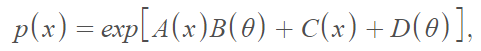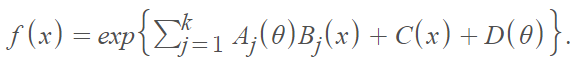# Darmois-Koopman Distribution

## What is the Darmois-Koopman Distribution?

A Darmois-Koopman distribution (or Koopman-Darmois) is a member of the exponential-type class of probability distributions. Exponential-type density functions have the formWhere A(·), B(·), C(·), and D(·) are arbitrary functions.

As a summation, the Darmois-Koopman distribution can be defined asWhere k is a set of jointly sufficient statistics for k parameters.

This class of probability distributions was recognized by Darmois  and Koopman  almost simultaneously . Pitman  is often credited with contributions to the introduction of the distribution. In both cases, the form of the PDF described a single sufficient statistic for θ, given n iid random variables. A broad subclass of these distributions was presented by Morris . The distribution remains relatively obscure, although it has made relatively recent appearances in estimation theory [7, 8].

## Darmois-Koopman-Pitman Theorem

The Darmois-Koopman-Pitman Theorem shows that sufficiency sharply restricts the form of the PDF. The theorem is related to the Darmois-Koopman distribution in the following way: given certain regularity conditions on the PDF, a necessary and sufficient condition for the existence of a sufficient statistic (possibly vector-valued) of fixed dimension is that the PDF is a member of the exponential distribution family .

## References

 Johnson, Kotz, and Balakrishnan, (1994), Continuous Univariate Distributions, Volumes I and II, 2nd. Ed., John Wiley and Sons.
 Commenges , D. et al. (Eds.). (2006). The Oxford Dictionary of Statistical Terms. Oxford University Press.
 Darmois, G. (1935). Sur les lois de probabilitis a estimation exhaustif, Compres Rendus de 1’Acadimie des Sciences, Paris, 200, 1265-1267.
 Koopman, B. 0. (1936). On distributions admitting a sufficient statistics, Transactions of the American Mathematical Society, 39, 339-409.
 Pitman (1936). Sufficient Statistics and Intrinsic Accuracy. Proc. Camb. Phi. Soc. 32: 567-569.
 Morris, C. N. (1983). Natural exponential families with quadratic variance function, Annals of Statistics, 11, 515-529.
 Kubacek, L. (2012). Foundations of Estimation Theory. Elsevier Science.
 American Mathematical Society (2008). Selected Papers on Analysis and Related Topics. American Mathematical Society.

CITE THIS AS:
Stephanie Glen. "Darmois-Koopman Distribution" From StatisticsHowTo.com: Elementary Statistics for the rest of us! https://www.statisticshowto.com/darmois-koopman-distribution/
---------------------------------------------------------------------------Need help with a homework or test question? With Chegg Study, you can get step-by-step solutions to your questions from an expert in the field. Your first 30 minutes with a Chegg tutor is free!